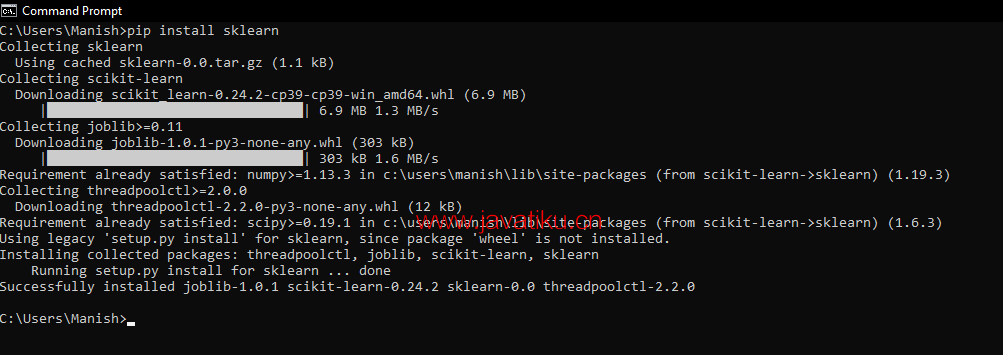# Tkinter 教程-Python SimpleImputer模块## SimpleImputer类

SimpleImputer类是scikit-learn库中的一个类，我们可以使用它来处理预测模型的数据中的缺失值。借助这个类，我们可以将数据集中的NaN（缺失值）值替换为指定的占位符。我们可以通过在程序中使用SimpleImputer()方法来实现和使用此模块类。

### SimpleImputer()方法的语法：

``SimpleImputer(missingValues, strategy)  ``

1. missingValues： 这是SimpleImputer()方法中的缺失值占位符，在执行过程中必须进行替代，默认情况下，缺失值占位符的值为NaN。
2. strategy： 这是将用于替换数据集中的缺失值（NaN值）的数据，并且默认情况下，此参数的值方法为'Mean'。SimpleImputer()方法的strategy参数可以接受'Mean'、'Mode'、'Median'（中心趋势测量方法）和'Constant'值输入。

SimpleImputer类是Sklearn库的模块类，要使用这个类，首先必须在系统中安装Sklearn库（如果尚未安装）。

### 安装Sklearn库：

``pip install sklearn  ``### 使用SimpleImputer类处理数据集中的NaN值

``````# Import numpy module as nmp
import numpy as nmp
# Importing SimpleImputer class from sklearn impute module
from sklearn.impute import SimpleImputer
# Setting up imputer function variable
imputerFunc = SimpleImputer(missing_values = nmp.nan, strategy ='mean')
# Defining a dataset
dataSet = [[32, nmp.nan, 34, 47], [17, nmp.nan, 71, 53], [19, 29, nmp.nan, 79], [nmp.nan, 31, 23, 37], [19, nmp.nan, 79, 53]]
# Print original dataset
print("The Original Dataset we defined in the program: \n", dataSet)
# Imputing dataset by replacing missing values
imputerFunc = imputerFunc.fit(dataSet)
dataSet2 = imputerFunc.transform(dataSet)
# Printing imputed dataset
print("The imputed dataset after replacing missing values from it: \n", dataSet2)  ``````

``````The Original Dataset we defined in the program:
[[32, nan, 34, 47], [17, nan, 71, 53], [19, 29, nan, 79], [nan, 31, 23, 37], [19, nan, 79, 53]]
The imputed dataset after replacing missing values from it:
[[32.   30.   34.   47.  ]
[17.   30.   71.   53.  ]
[19.   29.   51.75 79.  ]
[21.75 31.   23.   37.  ]
[19.   30.   79.   53.  ]]``````